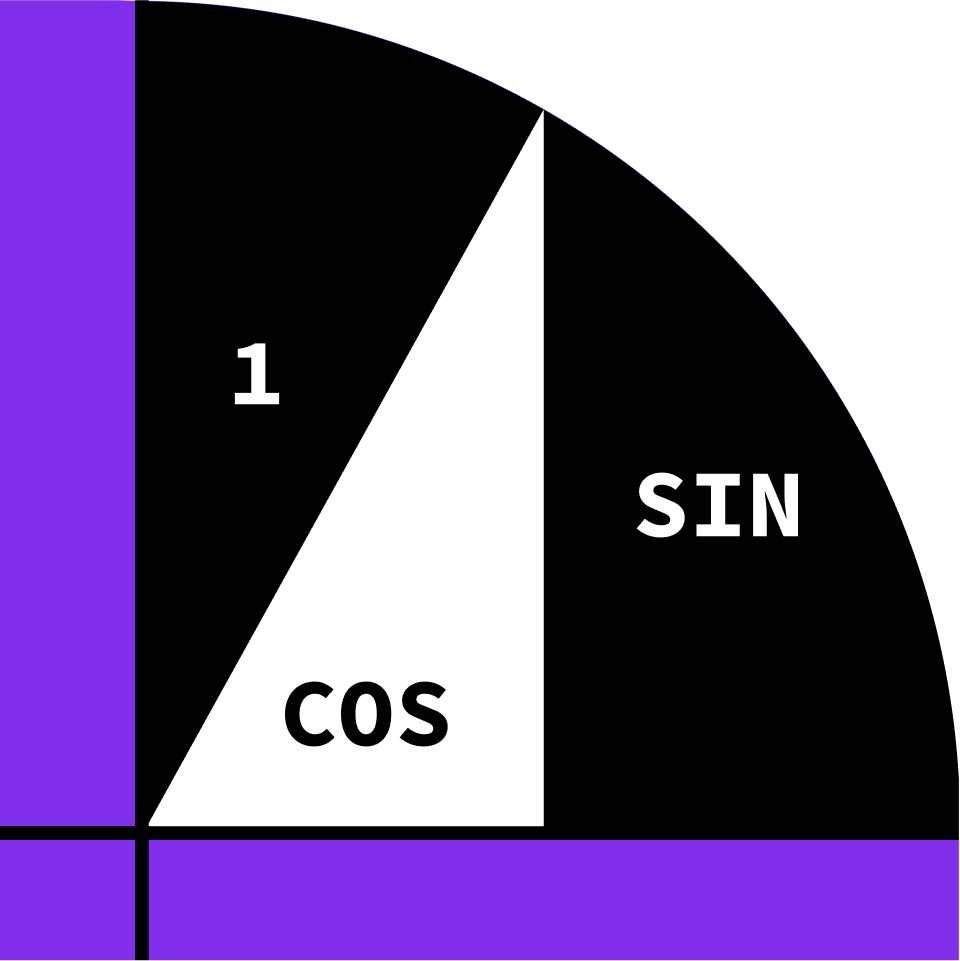## Calculus Fundamentals

Begin your calculus journey by getting up to speed on limits and derivatives.

Indeterminate Forms

Limits Intuition

Limits of Functions

Infinite Limits

Continuity

Computing Limits I

Computing Limits II

The Squeeze Theorem

IVT Application: Root Finder

Precise Definition of Limits

The Derivative at a Point

First Examples of Derivatives

What Derivatives Tell Us

The Second Derivative

Real-World Derivatives

Average vs. Instantaneous

Polynomials

Products, Reciprocals, and Quotients

Trigonometric Functions

The Chain Rule

Exponentials and Logarithms

Linear Approximation

Pendulums: An Application

Optimization

Implicit Differentiation

L'Hôpital's Rule

Antiderivatives & Integrals

### Course description

Calculus is the mathematical study of things that change: cars accelerating, planets moving around the sun, economies fluctuating. To study these changing quantities, a new set of tools - calculus - was developed in the 17th century, forever altering the course of math and science. This course sets you on the path to calculus fluency. The first part provides a firm intuitive understanding of limits, the central idea underlying the entire subject. The second part applies limits to define derivatives, an indispensable tool for measuring change. By the end of the course you'll have practical calculus experience that any aspiring scientist, engineer, or mathematician needs.

### Topics covered

• Continuity
• Derivatives
• Extrema
• Implicit Derivatives
• L'Hôpital's Rule
• Limits
• Newton's Method
• Physics Applications
• The Chain Rule
• The Squeeze Theorem

### Prerequisites and next steps

You’ll need an understanding of algebra and the basics of functions, such as domain and range, graphs, and intercepts. You should also be familiar with exponential functions, logarithms, and basic trigonometric identities.

• Trigonometry
• Pre-Calculus